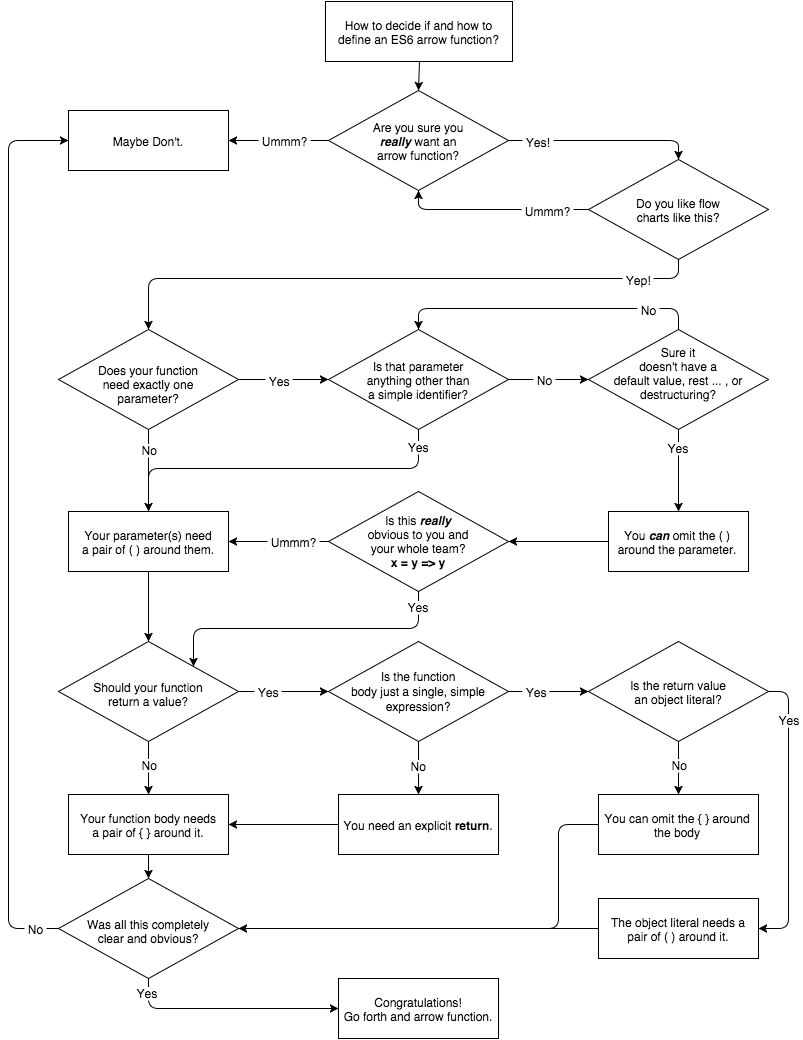## 箭头函数

function foo(x,y) {    return x + y;}// 对比var foo = (x,y) => x + y;

var f1 = () => 12;var f2 = x => x * 2;var f3 = (x,y) => {    var z = x * 2 + y;    y++;    x *= 3;    return (x + y + z) / 2;};

var a = [1,2,3,4,5];a = a.map( v => v * 2 );console.log( a );                // [2,4,6,8,10]

var dollabillsyall = (strings, ...values) =>    strings.reduce( (s,v,idx) => {        if (idx > 0) {            if (typeof values[idx-1] == "number") {                // look, also using interpolated                // string literals!                s += {values[idx-1].toFixed( 2 )};            }            else {                s += values[idx-1];            }        }        return s + v;    }, "" );

### 不只是简短的语法，而是this

var controller = {    makeRequest: function(..){        var self = this;        btn.addEventListener( "click", function(){            // ..            self.makeRequest(..);        }, false );    }};

var controller = {    makeRequest: function(..){        btn.addEventListener( "click", () => {            // ..            this.makeRequest(..);        }, false );    }};

var self = this（或者，另一种选择是，.bind(this)调用）通常可以帮忙的情况下，=>箭头函数是一个基于相同原则的很好的替代操作。听起来很棒，是吧？

var controller = {    makeRequest: (..) => {        // ..        this.helper(..);    },    helper: (..) => {        // ..    }};controller.makeRequest(..);

• 如果你有一个简短的，单语句内联函数表达式，它唯一的语句是某个计算后的值的return语句，并且 这个函数没有在它内部制造一个this引用，并且 没有自引用（递归，事件绑定/解除），并且 你合理地预期这个函数绝不会变得需要this引用或自引用，那么你就可能安全地将它重构为一个=>箭头函数。
• 如果你有一个内部函数表达式，它依赖于外围函数的var self = this黑科技或者.bind(this)调用来确保正确的this绑定，那么这个内部函数表达式就可能安全地变为一个=>箭头函数。
• 如果你有一个内部函数表达式，它依赖于外围函数的类似于var args = Array.prototype.slice.call(arguments)这样的东西来制造一个arguments的词法拷贝，那么这个内部函数就可能安全地变为一个=>箭头函数。
• 对于其他的所有东西 —— 普通函数声明，较长的多语句函数表达式，需要词法名称标识符进行自引用（递归等）的函数，和任何其他不符合前述性质的函数 —— 你就可能应当避免=>函数语法。